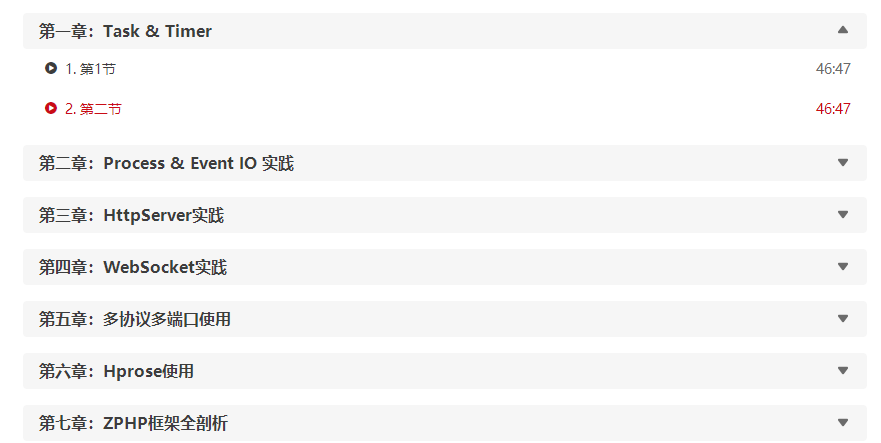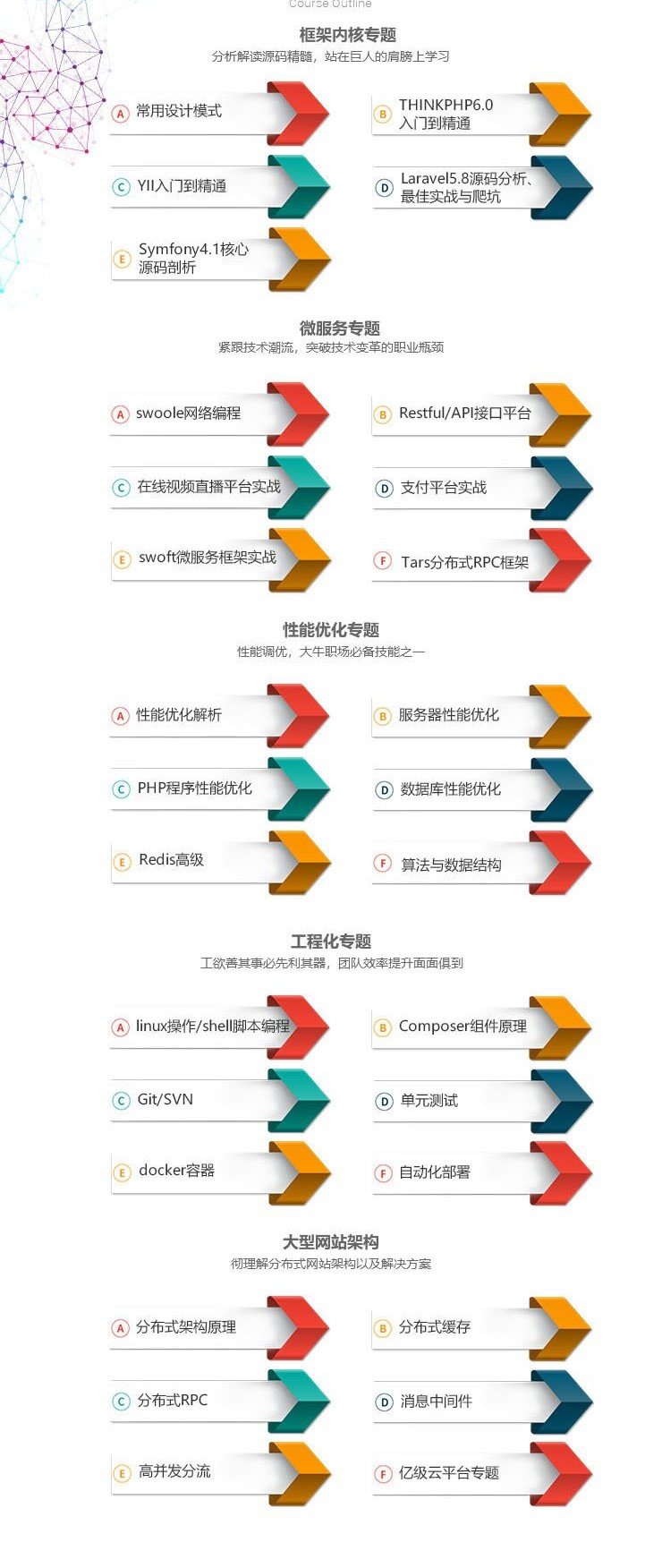﻿ 正确理解 PHP 的重载-综合-比特在线

### 正确理解 PHP 的重载

PHP 的重载跟 Java 的重载不同，不可混为一谈。Java 允许类中存在多个同名函数，每个函数的参数不相同，而 PHP 中只允许存在一个同名函数。例如，Java 的构造函数可以有多个，PHP 的构造函数则只能有一个。

PHP 的重载是指 通过魔术方法对属性和类的动态创建

● 属性的重载 - __get 与 __set

● 方法的重载 - __call 与 __callStatic

 1 `\$name` `= ``\$request``->name;`

 1 2 3 4 5 6 `public` `function` `__get(``\$key``)` `{` `    ``return` `Arr::get(``\$this``->all(), ``\$key``, ``function` `() ``use` `(``\$key``) {` `        ``return` `\$this``->route(``\$key``);` `    ``});` `}`

 1 2 3 4 5 6 7 8 9 10 11 12 13 14 15 16 17 18 19 20 21 22 23 24 `class` `Foo` `{   ` `    ``private` `\$params` `= [];` `    ``function` `__construct(``array` `\$params` `= [])` `    ``{` `        ``\$this``->params = ``\$params``;` `    ``}` `    ``public` `function` `__set(``\$name``, ``\$value``)` `    ``{` `        ``\$this``->params[``\$name``] = ``\$value``;` `    ``}` `    ``public` `function` `__get(``\$name``)` `    ``{` `        ``return` `\$this``->params[``\$name``];` `    ``}` `    ``public` `function` `__isset(``\$name``)` `    ``{` `        ``return` `isset(``\$this``->params[``\$name``]);` `    ``}` `    ``public` `function` `__unset(``\$name``)` `    ``{` `        ``unset(``\$this``->params[``\$name``]);` `    ``}` `}`明确的学习思路能更高效的学习